# Design of Fractional Delay FIR Filters

Fractional delay filters are aimed at shifting a digital sequence by a noninteger value, through interpolation and resampling combined into a single convolution filter. This example demonstrates the design and implementation of fractional delay FIR filters using tools available in the DSP System Toolbox™.

### Delay as a Convolution System

#### Integer Delays Delays

Consider the delay of a digital signal, $y\left[n\right]=x\left[n-D\right]$ where D is an integer. This operation can be represented as a convolution filter $y=h*x$, with a finite impulse response $\mathit{h}\left[\mathit{n}\right]=\delta \left[\mathit{n}-\mathit{D}\right]$. The corresponding transfer function is $H\left(z\right)={z}^{-D}$, and the frequeny response is $\mathit{H}\left(\omega \right)={\mathit{e}}^{-\mathit{i}\omega \text{\hspace{0.17em}}\mathit{D}}$. Programmatically, you can implement such an integer delay filter using the following MATLAB® code.

% Create the FIR D = 3; % Delay value h = [zeros(1,D) 1]
h = 1×4 0 0 0 1 

Shift a sequence by filtering it through the FIR h. Note the leading zeros at the beginning of the output, those signify the initial condition that is inherent to such filters.

x = (1:10)'; dfir = dsp.FIRFilter(h); y = dfir(x)'
y = 1×10 0 0 0 1 2 3 4 5 6 7 

#### Non-Integer Delays via D/A Interpolation

Delaying a sequence $\mathit{x}\left[\mathit{n}-\mathit{D}\right]$ is not defined whenever D is not an integer. To make such fractional delays sensible, one needs to add an intermediate D/A interpolation stage so as to sample the output on the continuum. That is, $\mathit{y}\left[\mathit{n}\right]=\stackrel{ˆ}{\text{\hspace{0.17em}}{\mathit{x}}_{\mathit{n}}}\left(\mathit{n}-\mathit{D}\right)$ where $\stackrel{ˆ}{\text{\hspace{0.17em}}{\mathit{x}}_{\mathit{n}}}$ denotes some D/A interpolation of the input sequence $\mathit{x}$. The D/A interpolating function$\stackrel{ˆ}{\text{\hspace{0.17em}}{\mathit{x}}_{\mathit{n}}}$ could depend on with n, and could be thought of as a representation of an underlying analog signal model from which the sequence $\mathit{x}$ was sampled. This strategy is used in other resampling problems such as rate conversion.

This example will feature fractional delay filters using two interpolation models, both of which are offered as a part of the DSP Systems Toolbox.

1. The sinc-based interpolation model, which uses a bandlimited reconstruction for $\stackrel{ˆ}{\text{\hspace{0.17em}}{\mathit{x}}_{\mathit{n}}}$.

2. The Lagrange-based interpolation model, which uses a polynomial reconstruction for $\stackrel{ˆ}{\text{\hspace{0.17em}}{\mathit{x}}_{\mathit{n}}}$.

#### Bandlimited Fractional Delay Filters

The Shannon-Whittaker interpolation formula $\stackrel{ˆ}{\text{\hspace{0.17em}}\mathit{x}}\left(\mathit{t}\right)=\sum _{\mathit{k}}\text{\hspace{0.17em}}\mathit{x}\left[\mathit{k}\right]\mathrm{sinc}\left(\mathit{t}-\mathit{k}\right)$ models bandlimited signals. That is, the intermediate D/A conversion $\stackrel{ˆ}{\text{\hspace{0.17em}}\mathit{x}}$ is a bandlimited reconstruction of the input sequence. For a delay value D, the fractional delay $\mathit{y}\left[\mathit{n}\right]=\stackrel{ˆ}{\text{\hspace{0.17em}}\mathit{x}}\left(\mathit{n}-\mathit{D}\right)$, which uses the same $\stackrel{ˆ}{\text{\hspace{0.17em}}\mathit{x}}$ for every n, can be represented as a convolution filter. This filter is called the ideal bandlimited fractional delay filter, and its impulse response is

${h}_{D}\left[k\right]=\text{sinc}\left(k-D\right)$.

The corresponding frequency response (that is, DTFT) is given by ${H}_{D}\left(\omega \right)={e}^{-i\omega D}$.

#### Causal FIR Approximation of the Ideal Bandlimited Shift Filter

The ideal sinc shift filter described in the previous section is an all-pass filter (i.e. $|{\mathit{H}}_{\mathit{d}}\left(\omega \right)|=1$), but it has an infinite and non-causal impulse response ${\mathit{h}}_{\mathit{D}}$. In MATLAB, it cannot be represented as a vector, but rather as a function of an index k.

% Ideal Filter sequence D = 0.4; hIdeal = @(k) sinc(k-D); 

For practical and computational purposes, the ideal filter can be truncated on a finite index window, at a cost of some bandwidth loss. For a target delay value of $\mathit{D}$ and a desired length of N, the window of indices $\mathit{k}\text{\hspace{0.17em}}$satisfying $|\mathit{D}-\mathit{k}|\le \frac{\mathit{N}}{2}$ is symmetric about $\mathit{D}$, and captures the main lobe of the ideal filter. For $\mathit{D}={\mathit{i}}_{0}+\mathit{FD}$ where $\mathit{0}\le \mathit{FD}\le 1$ and an integer ${\mathit{i}}_{0}$, the explicit window indices are$\left\{{i}_{0}-⌊\frac{N-1}{2}⌋,\dots ,\phantom{\rule{0.2em}{0ex}}{i}_{0}+⌊\frac{N}{2}⌋\right\}$. The integer ${\mathit{i}}_{0}$ is referred to as the integer latency, and can be chosen arbitrarily. To make the FIR causal, set ${\mathit{i}}_{0}=⌊\frac{\mathit{N}-1}{2}⌋$, so the index window is $\left\{0,\dots ,\mathit{N}-1\right\}$. The code below depicts the rationale behind the causal FIR approximation.

% FIR approximation with causal shift N = 6; idxWindow = (-floor((N-1)/2):floor(N/2))'; i0 = -idxWindow(1); % Causal latency hApprox = hIdeal(idxWindow); plot_causal_fir("sinc",D,N,i0,hApprox,hIdeal);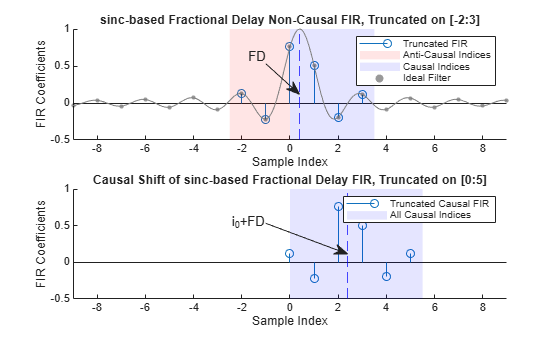Truncation of a sinc filter causes a ripple in the frequency response, which can be addressed by applying weights $\left\{{\mathit{w}}_{\mathit{k}}\right\}$ (such as Kaiser or Hamming) to the FIR coefficients .

Finally, the resulting FIR approximation model of the ideal bandlimited fractional delay filter is given below.

You can design such a filter using the designFracDelayFIR function and the dsp.VariableFractionalDelay System object™ in 'FIR' mode, both of which use Kaiser window weights.

#### Lagrange-based Fractional Delay Filters

Lagrange-based fractional delay filters use polynmial fitting on a moving window of input samples. That is, $\stackrel{ˆ}{\text{\hspace{0.17em}}{\mathit{x}}_{\mathit{n}}}\left(\mathit{t}\right)$ is a polynomial of some fixed degree K. Like the sinc-based delay filters, Lagrange-based delay filters can be formulated as a causal FIR convolution (i.e. $\mathit{y}=\mathit{h}*\mathit{x}$) of the length N=K+1, and supported on the index window$\left\{-⌊\frac{N-1}{2}⌋,\dots \phantom{\rule{0.2em}{0ex}}⌊\frac{N}{2}⌋\right\}$. Similarly to the sinc-based model, apply the causal latency ${\mathit{i}}_{0}=⌊\frac{\mathit{N}-1}{2}⌋$. Given a fractional delay $\mathit{FD}$, the FIR coefficients $\mathit{h}\left[0\right],\dots ,\mathit{h}\left[\mathit{K}\right]$ of the (causal shifted) Lagrange delay filter can be obtained by solving a system of linear equaions, as written below. Those equations describe a standard Lagrange polynomial fitting problem.

$\sum _{k=0}^{K}{t}_{n}^{k}h\left[k\right]=\left(FD{\right)}^{n},\phantom{\rule{0.2em}{0ex}}\phantom{\rule{0.2em}{0ex}}\phantom{\rule{0.2em}{0ex}}n=0,\dots ,\phantom{\rule{0.2em}{0ex}}K$

Here, ${\mathit{t}}_{0},\dots ,{\mathit{t}}_{\mathit{K}}$ are the enumerated indices of the sample window. The implementation is straightforward.

% Filter parameters FD = 0.4; K = 7; % Polynomial degree N = K+1; % FIR Length idxWindow = (-floor((N-1)/2):floor(N/2))'; % Define and solve Lagrange interpolation equations V = idxWindow.^(0:K); % Vandermonde structure C = FD.^(0:K); hLagrange = C/V; % Solve for the coefficients i0 = -idxWindow(1); % Causal latency plot_causal_fir("Lagrange",FD,N,i0,hLagrange);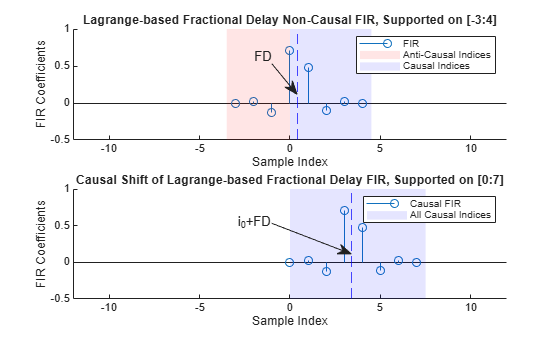This model can be implemented as a direct-form FIR filter if the delay value FD is fixed, or using a Farrow structure if the delay value is varying. There is a section below dedicated to the implementaiton of Lagrange interpolation using dsp.VariableFractionalDelay in 'Farrow' mode.

### Design And Implement sinc-Based Fractional Delay FIR Filters

The following section is focuses on designing and implementing sinc-based fractional delay filters.

#### The Function designFracDelayFIR in Length-based Design Mode

The function designFracDelayFIR provides a simple interface to design a fractional delay FIR filter of delay value FD and of length N.

FD = 0.32381; N = 10; h = designFracDelayFIR(FD,N)
h = 1×10 0.0046 -0.0221 0.0635 -0.1664 0.8198 0.3926 -0.1314 0.0552 -0.0200 0.0042 

The filter implementation can be done using any standard FIR filter, such as the dsp.FIRFilter System object.

% Create an FIR filter object fdfir = dsp.FIRFilter(h); 

Delay a signal by filtering it through the designed filter.

% Generate some input n = (1:100)'; x = gen_input_signal(n); % Filter the input signal y = fdfir(x); plot_sequences(n,x, n,y); legend('Filter Output','Original Sequence') title('Raw Filter Output v.s. Input Sequence')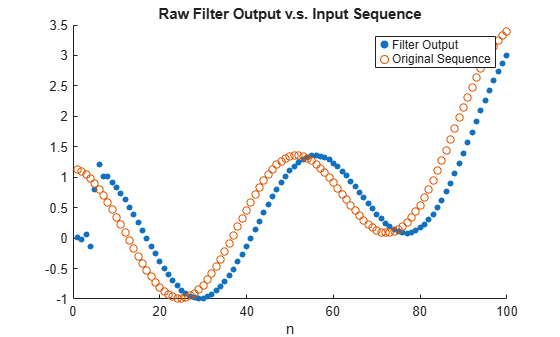Notice that the actual filter delay is not $\mathit{FD}$, but rather $\mathit{FD}+{\mathit{i}}_{0}$ because of the causal integer latency ${\mathit{i}}_{0}$. That latency is returned from the designFracDelayFIR function as a second output argument.

[h,i0] = designFracDelayFIR(FD,N);

The overall delay is merely the sum of the desired fractional delay and the incurred integer latency.

Dtotal = i0+FD
Dtotal = 4.3238 

This total delay is also the group delay of the FIR filter at low frequencies. Verify that by using the outputDelay function.

[Doutput,~,~] = fdfir.outputDelay(Fc=0)
Doutput = 4.3238 

Shift the plot of the input sequence by the total delay $\mathit{FD}+{\mathit{i}}_{0}$ to align the filter output with the expected result.

plot_sequences(n+Dtotal,x, n,y); legend('Filter Output','Input Sequence (shifted by FD+i0)') title('Filter Output v.s. Time Adjusted Input Sequence')Note that the shifted input markers located at $\left(\mathit{n}+\mathit{FD}+{\mathit{i}}_{0},\text{\hspace{0.17em}}\mathit{x}\left[\mathit{n}\right]\right)$ generally do not coincide with output samples markers $\left(\mathit{n},\text{\hspace{0.17em}}\mathit{y}\left[\mathit{n}\right]\right)$, because $\mathit{n}+\mathit{FD}+{\mathit{i}}_{0}$ falls on noninteger values on the x-axis, whereas n is integer. Rather, the shifted input samples fall approximately on a line connecting each two consecutive output samples.

plot_sequences(n+i0+FD,x, n,y,'with line'); legend('Filter Output','Input Sequence (shifted by FD+i0)') title('Output Samples v.s. Shifted Input Samples ') xlim([20,30])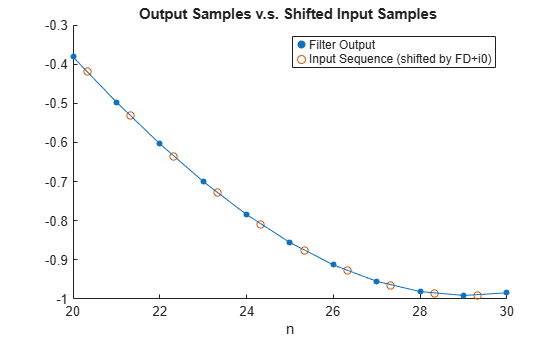#### The dsp.VariableFractionalDelay System Object in 'FIR' Mode

Similarly to designFracDelayFIR, the dsp.VariableFractionalDelay object can also design sinc-based delay filters when used with the 'FIR' inteprolation mode. Begin by creating an instance of the System object. The FIR length is always even, and is specified as a half-length parameter.

vfd_fir = dsp.VariableFractionalDelay(InterpolationMethod = 'FIR', FilterHalfLength = N/2); i0_vfd_fir = vfd_fir.FilterHalfLength; % Interger latency

Pass the desired fractional delay as the second input argument to the object call. Make sure that the delay value you specify includes the integer latency.

y = vfd_fir(x,i0+FD); release(vfd_fir) plot_sequences(n+i0+FD,x, n,y); legend('Filter Output','Input Sequence (shifted by FD+i0)') title('dsp.VariableFractionalDelay in FIR Mode')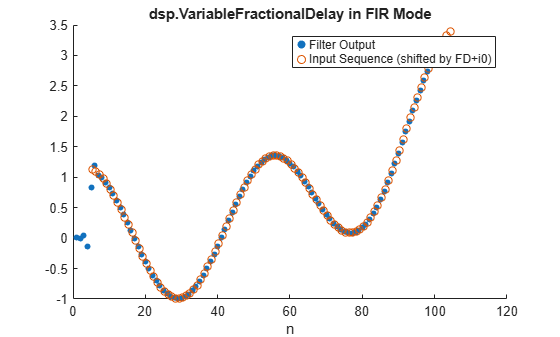#### Comparison of designFracDelayFIR and dsp.VariableFractionalDelay in 'FIR' Mode

Both designFracDelayFIR and dsp.VariableFractionalDelay in 'FIR' mode provide sinc-based fractional delay filters, but their implementations are different.

• The dsp.VariableFractionalDelay approximates the delay value by a rational number $\mathit{FD}\approx \mathit{k}}{\mathit{L}}$ up to some tolerance, and then samples the fractional delay as the k-th phase of a (long) interpolation filter of length L. This requires increased memory use, and yields less accurate delay.

• By contrast, designFracDelayFIR generates the FIR coefficients directly, rather than sampling them from a longer FIR. This gives the precise fractional delay value, and costs less memory.

• The designFracDelayFIR has a simple function interface returning the FIR coefficients, leaving the filter implementation to the the user. The dsp.VariableFractionalDelay is System object meant to encapsualte the filter design and implementation entirely.

The use of designFractionalDelayFIR is preferred over dsp.VariableFractionalDelay in 'FIR' mode for its simplicity, better performance, and efficiency. In the figure below, the filter designed with dsp.VariableFractionalDelay has a shorter bandwidth, and its group delay is off by ~0.02 from the nominal value.

% Obtain the FIR coefficients from the dsp.VariableFractionalDelay object h_vfd_fir = vfd_fir([1;zeros(31,1)],i0_vfd_fir+FD); release(vfd_fir); plot_freq_and_gd(h,i0,[],"designFracDelayFIR", h_vfd_fir,i0_vfd_fir,[],"dsp.VariableFractionalDelay FIR mode"); hold on; yline(FD,'DisplayName','Target Fractional Delay'); ylim([-0.1,0.4])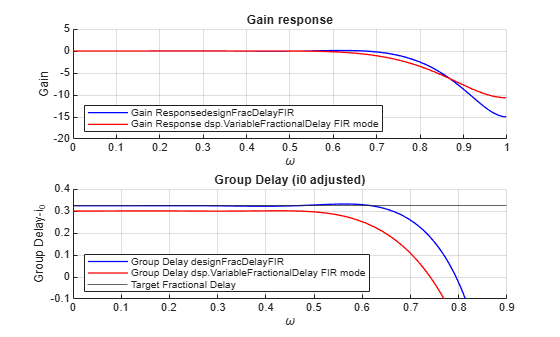### Design And Implement Lagrange-based Delay Filters

Lagrange-based fractional delay filters are computationally cheap and can be implemented efficiently using the Farrow structure. The Farrow filter is a special type of FIR that is implemented using only elementary algebraic operations, such as scalar additions and multiplications. Unlike the sinc-based designs, Farrow filters do not require specialized functions (such as sinc or Bessel) to compute the delay FIR coefficients. This makes Farrow fractional delay filters particularly simple to implement on a basic hardware.

On the downside, Lagrange-based delay filters are limited to low orders, due to the highly unstable nature of polynomial approximations of high degree. This usually results with a lower bandwidth, when compared with a sinc-based filter.

Another type of a computationally-cheap fractional delay filter is the Thiran filter. See thiran (Control System Toolbox) for more information.

#### The System Object dsp.VariableFractionalDelay in 'Farrow'mode

Use the system object dsp.VariableFractionalDelay in 'Farrow' mode to create and implement Farrow delay filters. Begin by creating an instance of the system object:

vfd = dsp.VariableFractionalDelay(InterpolationMethod = 'Farrow', FilterLength = 8); i0var = floor(vfd.FilterLength/2) % Interger latency of the filter
i0var = 4 

Apply the created object on the input signal, and plot the result.

y = vfd(x,i0var+FD); plot_sequences(n+i0var+FD,x, n,y); legend('Farrow Fractional Delay Output','Input Sequence (shifted by FD+i0)') title('dsp.VariableFractionalDelay in Farrow Mode')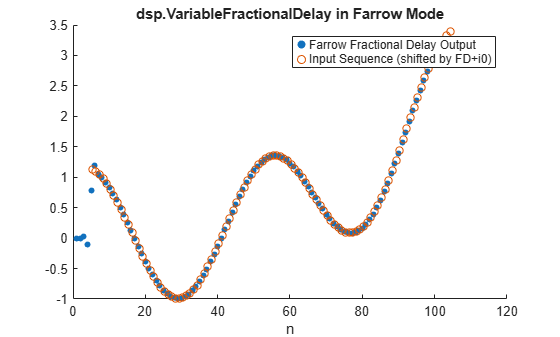You can also vary the fractional delay values. The code below operates on frames of 20 samples, while increasing the delay value with each frame. Note the increase of the delay in the output graph, corresponding to the changes in the delay values.

release(vfd) FDs = i0var+5*(0:0.2:0.8); % Fractional delays vector xsource = dsp.SignalSource(x,20); ysink = dsp.AsyncBuffer; for FD=FDs xk = xsource(); yk = vfd(xk, FD); write(ysink,yk); end y = read(ysink); plot_sequences(n+i0var,x, n,y); legend('Variable Fractional Delay Output','Original Sequence (shifted by i0)') title('dsp.VariableFractionalDelay in Farrow Mode, Varying Delay')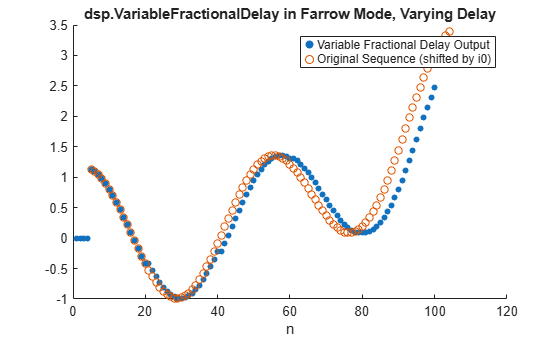### Bandwidth of FIR Fractional Delay Filters: Analysis and Design

Longer filters give better approximation of the ideal delay filter. Indeed, in terms of raw quadratic norms it is the case. However, we need a metric that is more practically meaningful, such as the bandwidth. The function designFracDelayFIR measures combined bandwidth, which is defined as the frequency range in which both the gain and the group delay are within 1% of their nominal values. The measured combined bandwidth can be obtained as a return value of the designFracDelayFIR function. Compare a filter of length 16 (blue) with a filter of length 256 (red) in the figure below. As expected, the longer filter have significantly higher combined bandwidth.

FD = 0.3; N1 = 16; N2 = 256; [h1,i1,bw1] = designFracDelayFIR(FD, N1); [h2,i2,bw2] = designFracDelayFIR(FD, N2); plot_freq_and_gd(h1,i1,bw1,"N="+num2str(N1), h2,i2,bw2,"N="+num2str(N2)); ylim([-0.2,0.6])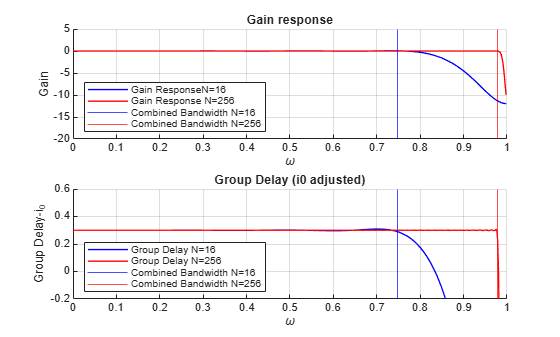#### The Function designFracDelayFIR in Bandwidth Design Mode

The bandwidth design mode of designFracDelayFIR can determine the required length for a given bandwidth. Specify the delay value and the desired target bandwidth as inputs to the function, and the function will find the appropriate length.

FD = 0.3; bwLower = 0.9; % Target bandwidth lower limit [h,i0fixed,bw] = designFracDelayFIR(FD,bwLower); fdfir = dsp.FIRFilter(h); info(fdfir)
ans = 6x35 char array 'Discrete-Time FIR Filter (real) ' '------------------------------- ' 'Filter Structure : Direct-Form FIR' 'Filter Length : 52 ' 'Stable : Yes ' 'Linear Phase : No ' 

Note that bwLower is merely a lower bound for the combined bandwidth. The function returns a filter whose combined bandwidth is at least the value specified in bwLow.

#### Distortion in High Bandwidth Signals

In this section, we compare the performance of the two design points (long sinc v.s. short Lagrange) with a high bandwidth input. The dsp.VariableFractionalDelay in the previous section is an 8-degree Farrow structure, effectively an FIR of length 9. The filter obtained by designFracDelayFIR(FD,0.9) has a length of 52 samples. Putting the two FIR frequency responses together on the same graph demonstrates the bandwidth difference between the two.

release(vfd); hvar = vfd([1;zeros(31,1)],i0var+FD); plot_freq_and_gd(h,i0fixed,bw,"Sinc-based", hvar,i0var,[],"Farrow"); ylim([-0.2,0.6])Apply the two filters on a high bandwidth signal, as compared in figure below. Sinc on the left column, Farrow on the right. Time domain on top row, frequency on the bottom. The results are, as expected:

• The longer sinc filter has a higher bandwidth. The shorter Farrow filter has lower bandwidth.

• Signal distortion is virtually nonexistent using the longer sinc filter, but easily noticeable in the shorter Farrow filter.

• The higher accuracy comes at the expense of longer latency: approximately 25 samples v.s. only 4 in the shorter filter.

n=(1:80)'; x = high_bw_signal(n); y1 = fdfir(x); y2 = vfd(x,i0var+FD); plot_signal_comparison(n,x,y1,y2,h,hvar,i0fixed,i0var,FD);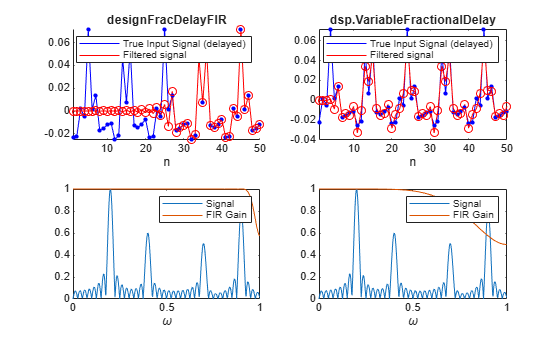### Which should be used: dsp.VariableFractionalDelay or designFracDelayFIR ?

This decision is largely based on filter requirements and the target platform.

• For a high bandwidth and accurate group delay response, use the designFracDelayFIR function. Keep in mind that this design process is more computationally intensive. Therefore, is it better suited to be deployed on a higher-end hardware, especially if realtime tuning of the delay value is desired. It is also suitable for lower-end hardware deployment, if the delay value is fixed, and the design can be done offline.

• For time-varying delay filters aimed at low-performance computational apparatus, use dsp.VariableFractionalDelay with the 'Farrow' mode.